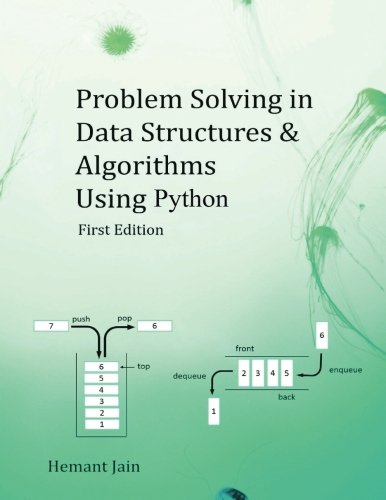# Problem Solving in Data Structures & Algorithms Using Python: Programming Interview Guide

Description

This book is about the usage of Data Structures and Algorithms in computer programming. Designing an efficient algorithm to solve a computer science problem is a skill of Computer programmer. This is the skill which tech companies like Google, Amazon, Microsoft, Adobe and many others are looking for in an interview. This book assumes that you are a Python language developer. You are not an expert in Python language, but you are well familiar with concepts of references, functions, lists and recursion. In the start of this book, we will be revising the Python language fundamentals. We will be looking into some of the problems in arrays and recursion too. Then in the coming chapter, we will be looking into complexity analysis. Then will look into the various data structures and their algorithms. We will be looking into a Linked List, Stack, Queue, Trees, Heap, Hash Table and Graphs. We will be looking into Sorting & Searching techniques. Then we will be looking into algorithm analysis, we will be looking into Brute Force algorithms, Greedy algorithms, Divide & Conquer algorithms, Dynamic Programming, Reduction, and Backtracking. In the end, we will be looking into System Design, which will give a systematic approach for solving the design problems in an Interview.

Chapter 0: How To Use This Book
Chapter 1: Introduction – Programming Overview
Chapter 2: Algorithms Analysis
Chapter 3: Approach To Solve Algorithm Design Problems
Chapter 4: Abstract Data Type & Python Collections
Chapter 5: Searching
Chapter 6: Sorting
Chapter 8: Stack
Chapter 9: Queue
Chapter 10: Tree
Chapter 11: Priority Queue
Chapter 12: Hash-Table
Chapter 13: Graphs
Chapter 14: String Algorithms
Chapter 15: Algorithm Design Techniques
Chapter 16: Brute Force Algorithm
Chapter 17: Greedy Algorithm
Chapter 18: Divide-And-Conquer, Decrease-And-Conquer
Chapter 19: Dynamic Programming
Chapter 20: Backtracking
Chapter 21: Complexity Theory And Np Completeness
Chapter 22: Interview Strategy
Chapter 23: System Design